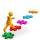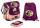Father and son

When I was 11, my father was 35 years old. Today, my father has three times more years than me. How old is she?

Result

j =  12
o =  36

Solution:

o = 3 j
o-j = 35 - 11

3j-o = 0
j-o = -24

j = 12
o = 36

Calculated by our linear equations calculator.

Leave us a comment of this math problem and its solution (i.e. if it is still somewhat unclear...):Be the first to comment!To solve this verbal math problem are needed these knowledge from mathematics:

Do you have a linear equation or system of equations and looking for its solution? Or do you have quadratic equation?

Next similar math problems:

1. Father and daughterWhen I was 11 my father was 35 year old. Now, father has three times older then me. How old is she?
2. Grandfather and grandmotherThe old mother is 5 years younger than the old father. Together they are 153 years old. How many years has each of them?
3. Family parcelsIn father will he divided the land so that the older son had three bigger part than younger son. Later elder son gave 2.5 ha field to younger and they had both the same. Determine the area of family parcel.
4. Journey to schoolOn the way to school I went steady step. In the first half of the journey, I counted every second step, in the second half every third. How many steps I do when I went to school if I counted double step are 250 more than three steps?
5. Ages 2A man's age is 4 times his son's age. After 5 years he will be just twice his son's age, find their ages.
6. Six yearsIn six years Jan will be twice as old as he was six years ago. How old is he?
7. SchoolsThree schools are attended by 678 pupils. To the first attend 21 students more and to the third 108 fewer students than to second school. How many students attend the schools?
8. The largerThe larger of two numbers is nine more than four times the smaller number. The sum of the two numbers is fifty-nine. Find the two numbers.
9. BarbaraBarborka goes to school with backpack that was 2 - times more expensive than a bag slipper. If backpack was 36 euros cheaper it was cost same as bag slipper. How many cost backpack and how many bas slipper?
10. Boys and girlsThere are 48 children in the sports club, boys are 10 more than girls. How many girls go to the club?
11. Two trainsThere were 159 freight wagons on the railway station creating 2 trains. One had 15 more wagons than the other. How many wagons did each train have?
12. Football match 4In a football match with the Italy lost 3 goals with Germans. Totally fell 5 goals in the match. Determine the number of goals of Italy and Germany.
13. Substitutionsolve equations by substitution: x+y= 11 y=5x-25
14. ATC campThe owner of the campsite offers 79 places in 22 cabins. How many of them are triple and quadruple?
15. Vijuviju has 40 chickens and rabbits. If in all there are 90 legs. How many rabbits are there with viju??
16. Belgium vs ItalyBelgium played a match with Italy and Belgium win by 2 goals. The match fell a total 6 goals. Determine the number of goals scored by Belgium and by Italy.
17. Linear systemSolve this linear system (two linear equations with two unknowns): x+y =36 19x+22y=720